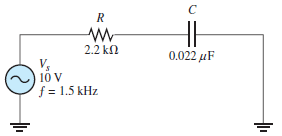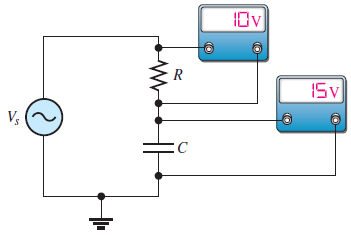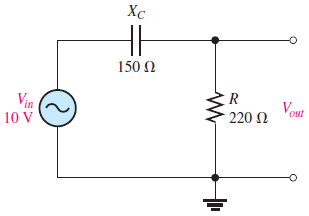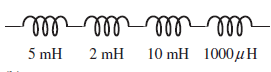# SSC JE Electrical Important MCQ Part 16

SSC JE Electrical Important MCQ PDF Part 16

1.The impedance of given circuit isA.110 Ω
B.100 Ω
C.53 Ω
D.147 Ω

2.The capacitive reactance of given circuit isA.5.82 kΩ
B.4.82 kΩ
C.82.4 kΩ
D.82.5 kΩ

3.The source voltage Vs of given circuit isA.18 V
B.25 V
C.5 V
D.16 V

Answer  : A (Since Vr and Vc are 90⁰ out of phase,we cannot add them directly. The source voltage is the phasor sum of Vr and Vc, Vs =√Vr²+Vc² =√10²+15²=18 V)

4.The output voltage Vout of given circuit isA.8.26 V
B.2.5 V
C.5 V
D.1.62 V

Answer  : A (Vout =(R/√R²+Xc²)×Vin =(220√200²+150²)×10=8.26 V)

5.Phase angle φ of lead circuit is given by

A.tan⁻¹(Xc/R)
B.tan⁻¹(R/Xc)
C.tan⁻¹(R)
D.tan⁻¹(Xc)

6.Total inductance of the series connections in FigureA.18 μH
B.18 H
C.18 mH
D.1.8 H

Answer  : C (L total = 5mH + 2mH + 10 mH + 1mH = 18 mH, Note-1000 μH= 1mH)

7.A series RL circuit has a resistance of 1.0 kΩ and an inductance of 1.0 mH. What is the time constant

A.1.9 μs
B.1.0 μs
C.1.9 s
D.1.0 s

Answer  : B (τ=L/R=1.0 mH/1.0 kΩ=1×10⁻³ H/1×10³ Ω=1.0 ×10 ⁻⁶s=1.0 μs)

8.Three loads, each of resistance 30Ω , are connected in star to a 415V, 3-phase supply.The system phase voltage is

A.415V
B.316V
C.220V
D.240V

Answer  : D (For a star connection, VL =√3 Vp. Hence phase voltage, Vp =VL/√3=415/√3=239.6V or 240V)

9.A transformer having a turns ratio of 4:1 supplies a load of resistance 100Ω.The equivalent input resistance of the transformer is

A.400 Ω
B.200 Ω
C.800 Ω
D.1600 Ω

Answer  : D (The equivalent input resistance,R₁=(N₁/N₂)²R₂=(4/1)²×100=1600Ω)

10.A generator is connected to a 60Ω load and a current of 8A flows. If the armature resistance is 1Ω,Then generated e.m.f. is

A.480 V
B.444 V
C.488 V
D.490 V

(Terminal voltage, V=IaRL =(8)(60)=480 volts
Generated e.m.f.,
E = V + IaRa
= 480 + (8)(1) = 480 + 8 = 488 volts)

11.Which is the passive component in terms of electronics

A.Resistor
B.SCR
C.Transistor
D.Diode

12.How many number of parallel paths will be in simplex 4 pole wave winding

A.4
B.8
C.2
D.6

Answer  : C (The number of parallel paths is equal to two always in wave winding)

13.Skin effect in a conductor becomes more pronounced ___________

A.at lower frequency
B.at DC voltage
C.at Ramp voltage
D.at high frequency

Answer  : D (Skin effect increases with the increase in frequency)

14.In three point starter, the overload release coil operates, if ___________ current increases beyond set limit.

A.Armature
B.Field
C.Line
D.Neutral

15.What is the full form of LPMV lamps

A.Low Potential Mercury Vapour lamps
B.Low Pressure Mercury Vapour lamps
C.Low Pressure Metal Voltage lamps
D.Low pressure Mercury Voltage lamps

16.What is the purpose of armouring in the UG cable

A.Protect the cable from entering of water
B.Insulate the sheath of the cable.
C.Protect the cable from mechanical injury
D.Protect the cable from corrosion

Answer  :  C (Armoring is provided over the bedding which consists of one or two layers of galvanized steel wire or steel tape. It protects the core from mechanical injury)

17.A SCR is a ______________ switch.

A.Four directional
B.Unidirectional
C.Three directional
D.Bidirectional

18.What is the full form of RCC poles

A.Reinforced Concrete column
B.Residual Cement Column
C.Reinforced Cement Column
D.Reinforced Cement concrete

19.As per the IE rules, the maximum load on the power sub-circuit should be restricted to —-.

A.1000 Watts
B.800 Watts
C.3000 Watts
D.5000 Watts

Answer  :  C (The load connected in a power sub-circuit normally should not exceed 3,000 watts)

20.When the 3 phase power is measured by two wattmeter method, at what power factor, the readings of both wattmeter will be positive but not equal

A.Between unity to 0.5 lag
B.At 0.5 Lag
C.Between 0.5 lag to zero
D.At Unity

Answer  :  A (When the power factor of load is unity then both the wattmeter reads the same value & when power factor of load is 0.5 lagging then power is only measured by first wattmeter and reading of second wattmeter is zero,Hence Between unity to 0.5 lag the readings of both wattmeter will be positive but not equal)